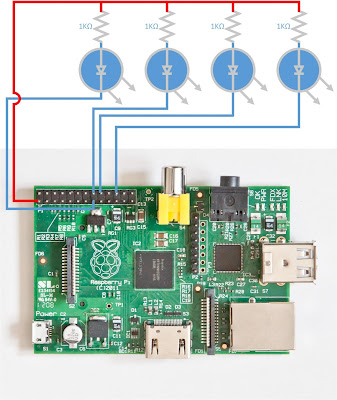### LED Blinky in Lua on Raspberry Pi running Mihini on ArchLinux

After setting up Eclipse M2M toolchain for Raspberry Pi, I wanted to try out a simple lua script to blink LEDs using GPIOs. Here is the schematic and lua script for the same. I used the 3.3V available on RPi for powering the LEDs. 1 kiloohm resistors were used to limit the current passing through the LED. The cathodes of the LED were connected to drain the current into the GPIO pins. I used 4 LEDs connected to 4 different GPIOS (GPIO 22, GPIO 23, GPIO 24, GPIO 25).SchematicPhoto of the setup, I used a LED/Switches widget

 ``` 1 2 3 4 5 6 7 8 9 10 11 12 13 14 15 16 17 18 19 20 21 22 23 24 25 26 27 28 29 30 31 32 33 34 35 36 37 38 39 40 41``` ```package.path = '/opt/mihini/lua/?.lua;/opt/mihini/lua/?/init.lua;' .. package.path package.cpath = '/opt/mihini/lua/?.so;' .. package.cpath local gpio = require"gpio" local clock = os.clock local function sleep(n) -- seconds local t0 = clock() while clock() - t0 <= n do end end local function toggle_pin(n) local stat = gpio.read(n) if stat == 1 then gpio.write(n,0) elseif stat == 0 then gpio.write(n,1) end end local function main() gpio.configure(22, {direction="out", edge="both", activelow="0"}) gpio.configure(23, {direction="out", edge="both", activelow="0"}) gpio.configure(24, {direction="out", edge="both", activelow="0"}) gpio.configure(25, {direction="out", edge="both", activelow="0"}) gpio.write(22, 1) gpio.write(23, 1) gpio.write(24, 1) gpio.write(25, 1) while true do sleep(1) toggle_pin(22) toggle_pin(23) toggle_pin(24) toggle_pin(25) end end main() ```
Lua script code to blink 4 LEDs

References: